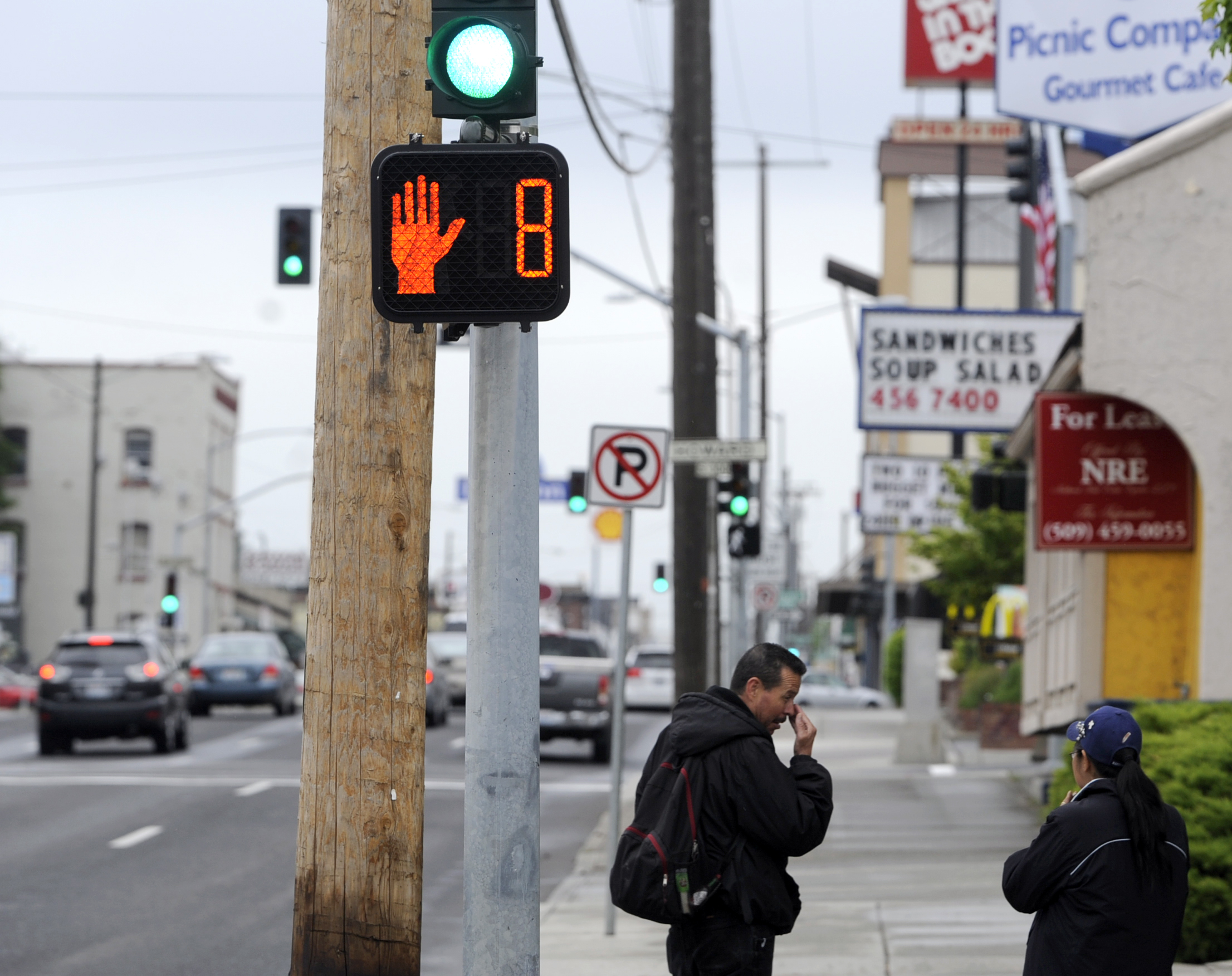## Timing a Crosswalk

A traffic controller is installing a brand new pedestrian crosswalk at a busy urban intersection. The default configuration displays the initial "WALK" signal for 5.0 seconds. Then, the flashing "DON'T WALK" signal is displayed immediately after, before it stops flashing. If the average pedestrian walking speed is 1.5 meters/second, and the crosswalk length is 10 meters wide, how long should the crosswalk remain "green" to allow a safe pedestrian crossing?Hint
$$Green=WALK+\frac{width}{pedestrian\:speed}$$$$$Green=WALK+\frac{width}{pedestrian\:speed}$$$
$$=5s+\frac{10m}{1.5m/s}=11.67\:seconds$$$$$=11.67\:seconds$$$